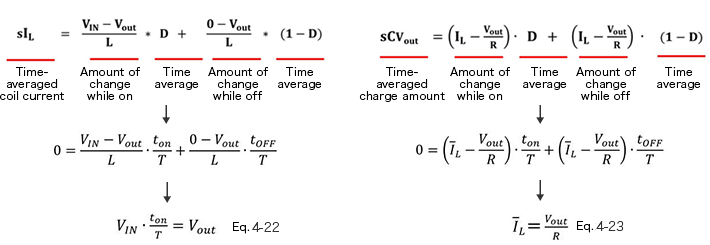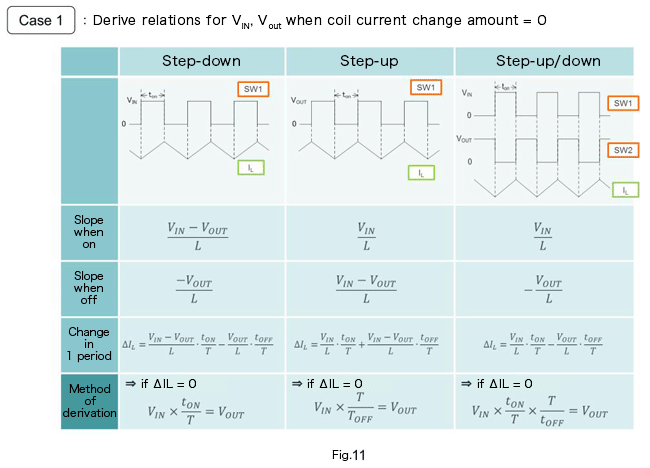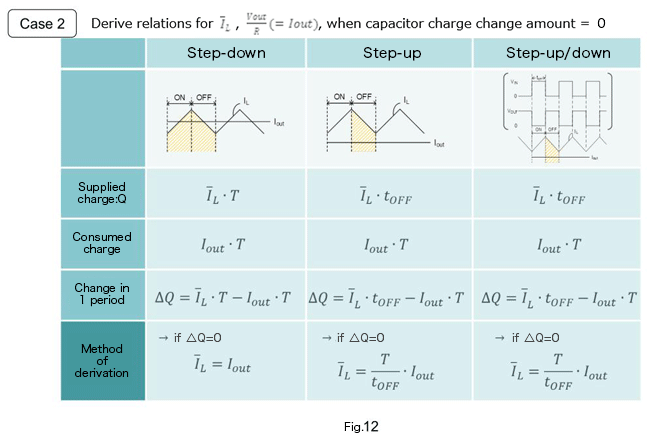Technical Information Site of Power Supply Design

2017.08.24 Transfer Function

# Switching Transfer Functions: State-Space Averaging Method － Static Analysis

DC/DC Converters: Sharing of Transfer Functions among Control Systems

In the previous section, we explained "state-space averaging" in order to derive a transfer function that could be applied to step-down, step-up, and step-up/down modes. This time, we perform a static analysis based on the derived equations. The equations of the previous section are used, and so it may aid understanding to read the contents of the previous section as well.

Static Analysis

The equations derived the previous time for coil current and capacitor charge amount are shown on the first line.

We perform a static analysis based on these equations. In a static analysis (steady state/stable state), the amount of change in the coil current and the amount of change in the charge on the output capacitor are both 0 (zero), and therefore the equations with 0 substituted are shown on the second line. D is rewritten as the time.

Rearranging these equations, we obtain equations 4-22 and 4-23 on the third line.Equation 4-22 is the well-known relation betweenVIN, Vout and D.

Equation 4-23 means that the average value of the coil current is supplied to the output load in one period.

This approach can be applied not only to step-down converters, but to step-up converters and step-up/down converters as well.

Below, static-state operation of the different converter types is summarized.This website uses cookies.

By continuing to browse this website without changing your web-browser cookie settings, you are agreeing to our use of cookies.